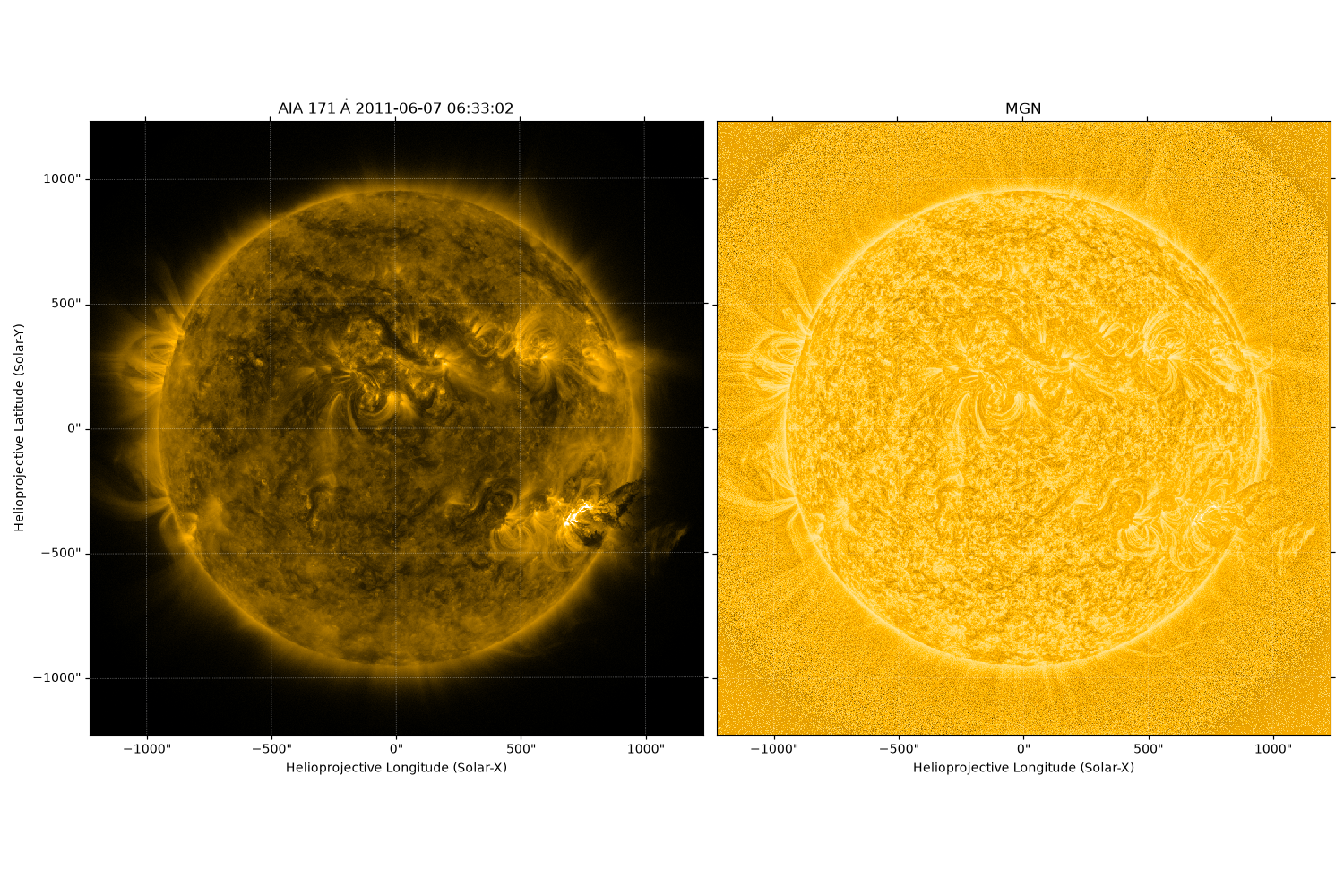# Multi-scale Gaussian Normalization#

This example applies Multi-scale Gaussian Normalization to a SunPy Map using sunkit_image.enhance.mgn.

import matplotlib.pyplot as plt
import sunpy.data.sample
import sunpy.map

import sunkit_image.enhance as enhance


SunPy sample data contains a number of suitable images, which we will use here.

aia_map = sunpy.map.Map(sunpy.data.sample.AIA_171_IMAGE)

# The original image is plotted to showcase the difference.
fig = plt.figure()
ax = plt.subplot(projection=aia_map)
aia_map.plot()Files Downloaded:   0%|          | 0/1 [00:00<?, ?file/s]

AIA20110607_063302_0171_lowres.fits:   0%|          | 0.00/973k [00:00<?, ?B/s]

AIA20110607_063302_0171_lowres.fits:  60%|██████    | 585k/973k [00:00<00:00, 5.11MB/s]

<matplotlib.image.AxesImage object at 0x7f64bebeb940>


Applying Multi-scale Gaussian Normalization on a solar image. The sunkit_image.enhance.mgn function takes a numpy.ndarray as a input so we will pass only the data part of GenericMap

out = enhance.mgn(aia_map.data)
# The value returned is also a numpy.ndarray so we convert it back to
# a  sunpy.map.GenericMap.
out = sunpy.map.Map(out, aia_map.meta)


Now we will plot the final result.

fig = plt.figure()
ax = plt.subplot(projection=out)
out.plot()

plt.show()Total running time of the script: (0 minutes 3.841 seconds)

Gallery generated by Sphinx-Gallery